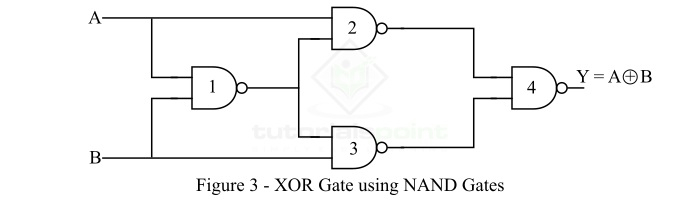# Implementation of XOR Gate from NAND Gate

The NAND Gate is a universal logic gate, using which we can implement any other type of logic gate or logical expression. Read this tutorial to understand how you can implement a XOR gate using only NAND gates. Let's start with a basic overview of XOR and NAND gates

## What is a XOR Gate?

The XOR (Exclusive-OR) Gate is a type of derived logic gate. The XOR gate is a logic gate that has two inputs and one output. The XOR gate produces a HIGH (Logic 1) output when one and only one of its two inputs are HIGH (Logic 1). When both inputs of the XOR gate are HIGH (Logic 1) or LOW (Logic 0), then the output of the XOR gate is a LOW (Logic 0) state. The logic symbol of the XOR gate a is shown in Figure-1.Hence, the XOR gate produces an output HIGH only when its inputs are not equal. Therefore, the XOR gate is also known as "anti-coincidence gate" or "inequality detector".

The output of the XOR gate is the modulo sum of its inputs, i.e.,

$$\mathrm{Y=A\bigoplus B=A\bar{B}+\bar{A}B}$$

Where, A and B are the two input variables to the XOR gate, Y is the output variable of the XOR gate. The output expression of the XOR gate is read as Y is equal to A ex-or B.

### Truth Table of XOR Gate

The truth table shows the relationship between inputs and output of the XOR gate. The truth table of an XOR gate is shown below.

Input

Output

A

B

Y = (AB' +A'B)

0

0

0

0

1

1

1

0

1

1

1

0

## What is a NAND Gate?

A NAND Gate is a type of universal logic gate that can be used to realize any kind logical expression or any other type of logic gate. A NAND gate is basically a combination of two basic logic gates namely, AND gate and NOT gate, i.e.,

$$\mathrm{NAND\:Logic=AND\:Logic=NOT\:Logic}$$

A NAND gate is the type of logic gate whose output is LOW (Logic 0) when all its inputs are high, and its output is HIGH (Logic 1), when any of its inputs is LOW (Logic 0). Therefore, the operation of the NAND gate is opposite that of the AND gate. The logic symbol of a two input NAND gate is shown in Figure-2.### Output Equation of NAND Gate

If A and B are the input variables and Y is the output variable of the NAND gate, then its output is given by

$$\mathrm{Y=\overline{A\cdot B}=(A\cdot B)'}$$

It is read as "Y is equal to A.B whole bar".

### Truth Table of NAND Gate

The following is the truth table of the NAND gate:

Input

Output

A

B

Y = (A.B)'

0

0

1

0

1

1

1

0

1

1

1

0

Now, let us discuss the implementation of XOR Gate from NAND Gate.

## Implementation of XOR Gate from NAND Gate

As discussed above, the NAND gate is a universal logic, hence, using which we may implement any other logic gate. Figure-3 shows how you can implement a XOR gate using only NAND gates.From the logic circuit diagram of the XOR gate using NAND gates only, it is clear that we require 4 NAND gates.

Now, let us understand how this NAND logic circuit functions to produce an output equivalent to the XOR gate.

The output of the first NAND gate is,

$$\mathrm{Y_1=\overline{A B}}$$

The outputs of the secondary and third NAND gates are,

$$\mathrm{Y_2=\overline{A\cdot \overline{AB}}}$$

$$\mathrm{Y_3=\overline{B\cdot \overline{AB}}}$$

Finally, these two outputs (Y2 and Y3) are connected to the fourth NAND gate. This NAND gate will produce an output which is,

$$\mathrm{Y=\overline{\overline{A\cdot \overline{AB}}\cdot \overline{B\cdot\overline{AB}}}}$$

$$\mathrm{\Rightarrow Y=A\cdot\overline{AB}+B\cdot\overline{AB}=A(\bar{A}+\bar{B})+B(\bar{A}+\bar{B})}$$

$$\mathrm{\Rightarrow Y=A\bar{A}+A\bar{B}+\bar{A}B+B\bar{B}}$$

$$\mathrm{\therefore Y=A\bar{B}+\bar{A}B = A\bigoplus B}$$

This is the output of the XOR gate. Hence, in this way, we can implement the XOR gate from NAND gates only.

Updated on: 14-Sep-2023

30K+ Views